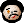# 2022-08-22 The Emacs range package

Some time ago I discovered something really cool. It turns out Emacs has a package named `range` which can be used to operate on, well, ranges of integers. The truth is, it supports much more: arbitrary finite sets of integers.

For example, you can use the function `range-compress-list` to convert a (sorted) list of integers into a “compact” range notation:

```(range-compress-list '(1 2 3 5 6 7 10 15 16))
;; => ((1 . 3) (5 . 7) 10 (15 . 16))
```

Of course, you can also perform the opposite conversion:

```(range-uncompress '((1 . 3) (5 . 7) 10 (15 . 16)))
;; => (1 2 3 5 6 7 10 15 16)
```

The cool thing is that there is a set of set-theoretic operations implemented there (pun intended!), though one of them is named in a bit unexpected way:

```(range-member-p 4 '((1 . 3) (5 . 7)))
;; => nil
(range-concat '((1 . 3) (5 . 7)) '((2 . 6)))
;; => ((1 . 7))
(range-difference '((1 . 10)) '((4 . 6)))
;; => ((1 . 3) (7 . 10))
(range-intersection '((1 . 3) (5 . 7)) '((3 . 8)))
;; => (3 (5 . 7))
```

I have no idea if I will ever use this. The only use-case I can think of would be to say something like `(range-uncompress '((1 . 10)))` to get `(1 2 3 4 5 6 7 8 9 10)` – but this can be done without `require`​’ing `range`, using `(number-sequence 1 10)`. Still, `range` looks really cool! (If you are wondering, the only place in Emacs code where `range` is actually used seems to be Gnus, and I didn’t look how exactly it is used – Gnus is a huge beast, I wasn’t brave enough to dive into its code.)

I suppose the bottom line is that you can find real gems in Emacs sources if you look in the right place. Like I didn’t know that already…